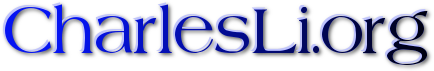# My notes

• Trace formula on hyperbolic plane: Trace formulas were introduced by Selberg in 1956. The original setting used differential geometry. Most of the modern settings use representation theory. In this slide, we will discuss Selberg's original approach. We will derive Selberg trace formula on compact hyperbolic surface using modern setting.
• Fourier coefficient of modular forms and the Petersson Formula: This is a note written by Don Larson. It came out from an informal reading course conducted by Don and me. Our main goals were to derive the standard bounds on the size of Fourier coefficients of cusp forms (leading up to the Ramanujan conjectures) and to prove the Petersson formula.